Publicité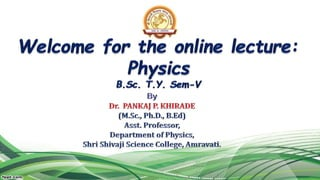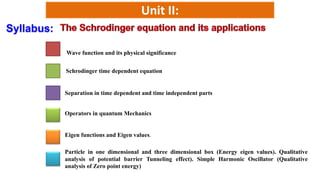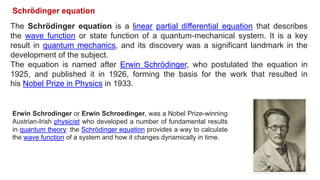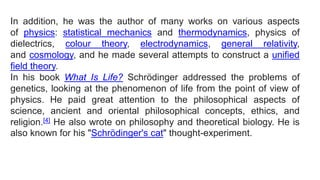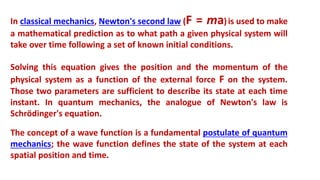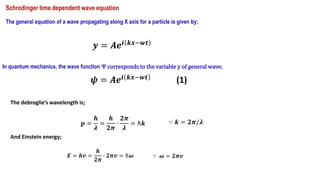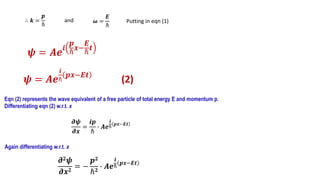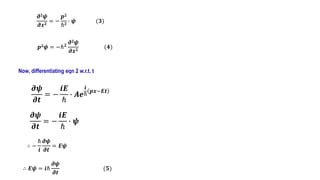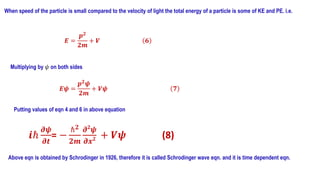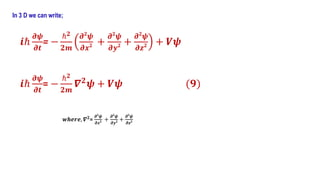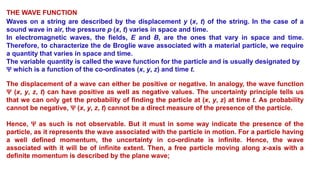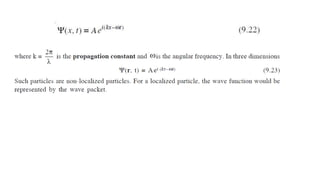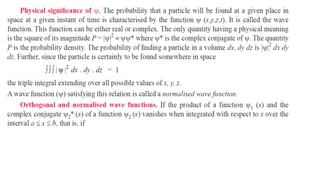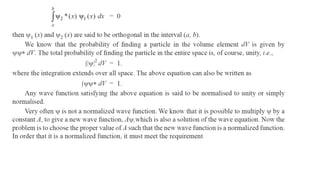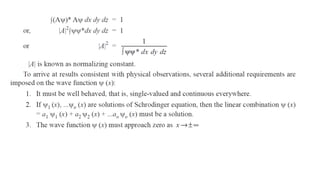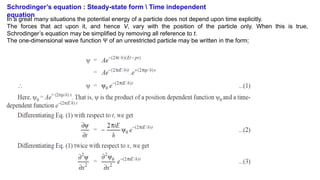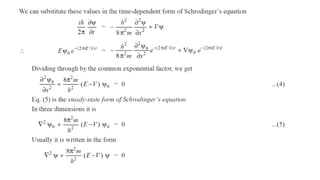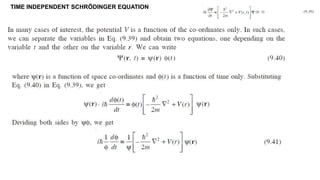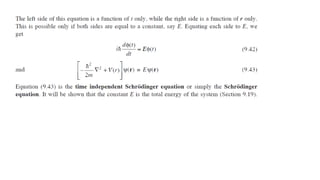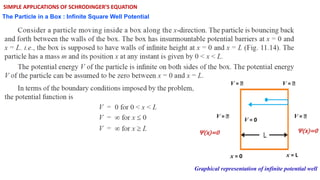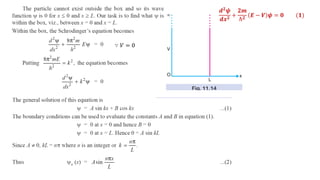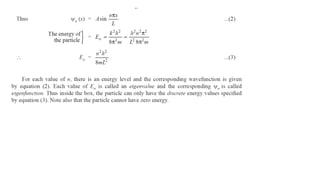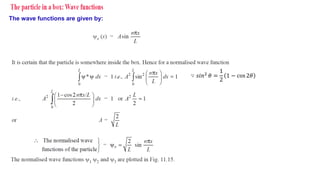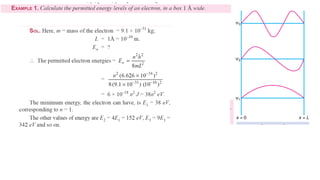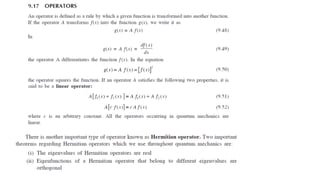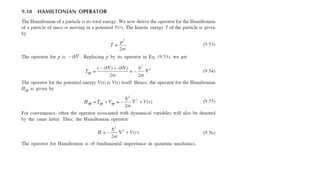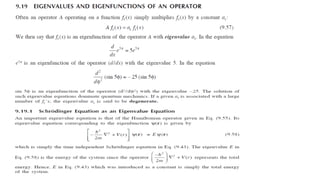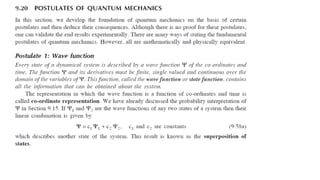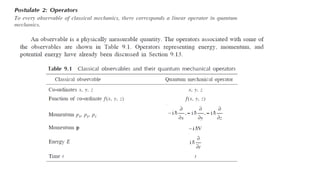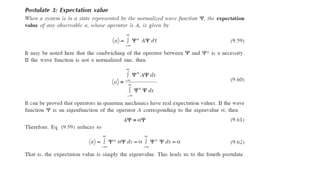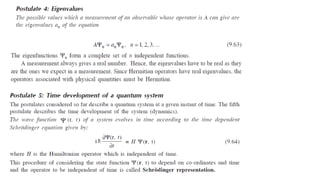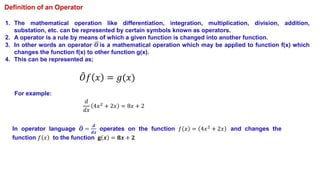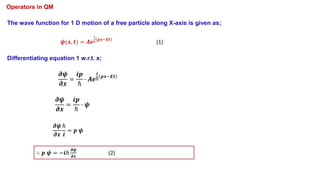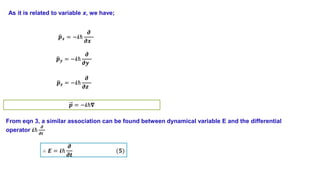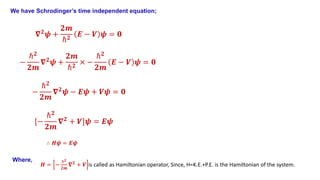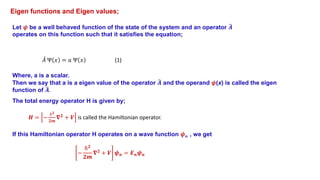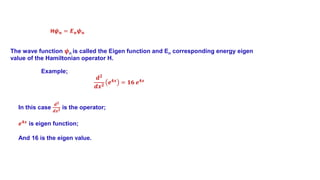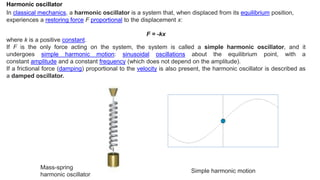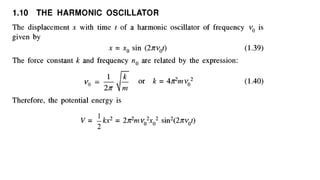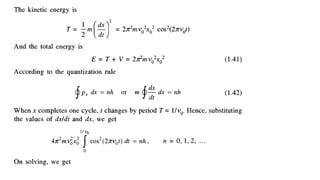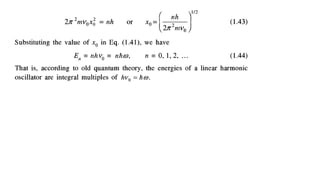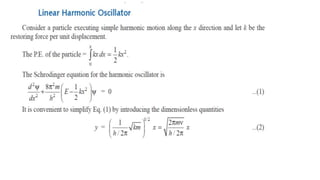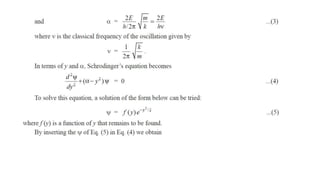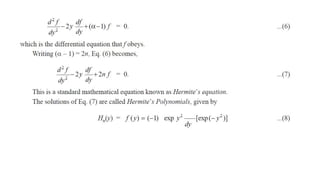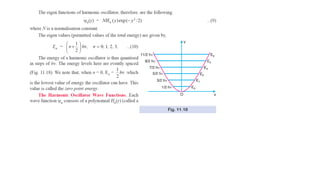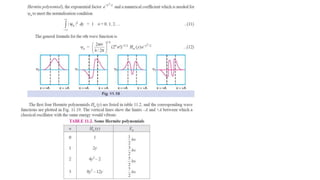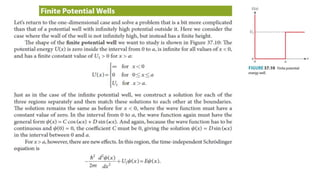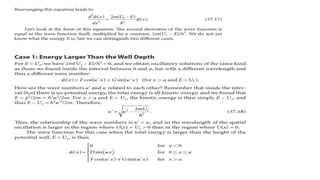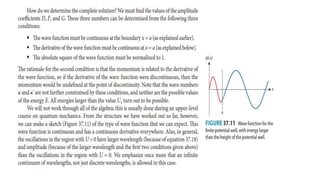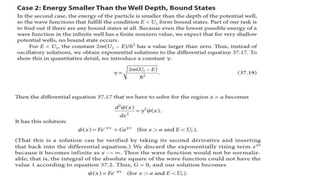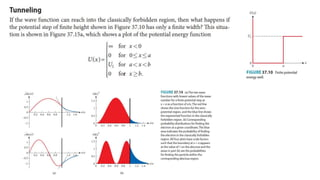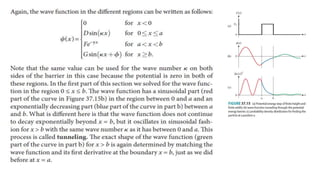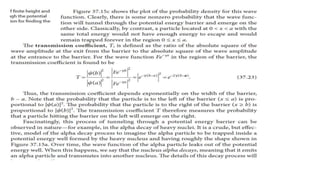1 sur 56
Publicité

### Schrodinger equation and its applications: Chapter 2

1. Unit II: Wave function and its physical significance Schrodinger time dependent equation Separation in time dependent and time independent parts Operators in quantum Mechanics Eigen functions and Eigen values. Particle in one dimensional and three dimensional box (Energy eigen values). Qualitative analysis of potential barrier Tunneling effect). Simple Harmonic Oscillator (Qualitative analysis of Zero point energy) Syllabus:
2. The Schrödinger equation is a linear partial differential equation that describes the wave function or state function of a quantum-mechanical system. It is a key result in quantum mechanics, and its discovery was a significant landmark in the development of the subject. The equation is named after Erwin Schrödinger, who postulated the equation in 1925, and published it in 1926, forming the basis for the work that resulted in his Nobel Prize in Physics in 1933. Erwin Schrodinger or Erwin Schroedinger, was a Nobel Prize-winning Austrian-Irish physicist who developed a number of fundamental results in quantum theory: the Schrödinger equation provides a way to calculate the wave function of a system and how it changes dynamically in time. Schrödinger equation
3. In addition, he was the author of many works on various aspects of physics: statistical mechanics and thermodynamics, physics of dielectrics, colour theory, electrodynamics, general relativity, and cosmology, and he made several attempts to construct a unified field theory. In his book What Is Life? Schrödinger addressed the problems of genetics, looking at the phenomenon of life from the point of view of physics. He paid great attention to the philosophical aspects of science, ancient and oriental philosophical concepts, ethics, and religion. He also wrote on philosophy and theoretical biology. He is also known for his "Schrödinger's cat" thought-experiment.
4. In classical mechanics, Newton's second law (F = ma)is used to make a mathematical prediction as to what path a given physical system will take over time following a set of known initial conditions. Solving this equation gives the position and the momentum of the physical system as a function of the external force F on the system. Those two parameters are sufficient to describe its state at each time instant. In quantum mechanics, the analogue of Newton's law is Schrödinger's equation. The concept of a wave function is a fundamental postulate of quantum mechanics; the wave function defines the state of the system at each spatial position and time.
5. Schrodinger time dependent wave equation The general equation of a wave propagating along X axis for a particle is given by; 𝒚 = 𝑨𝒆𝒊 𝒌𝒙−𝒘𝒕 In quantum mechanics, the wave function Ψ corresponds to the variable y of general wave; 𝝍 = 𝑨𝒆𝒊 𝒌𝒙−𝒘𝒕 (1) The debroglie’s wavelength is; 𝒑 = 𝒉 𝝀 = 𝒉 𝟐𝝅 ∙ 𝟐𝝅 𝝀 = ℏ𝒌 And Einstein energy; 𝑬 = 𝒉𝒗 = 𝒉 𝟐𝝅 ∙ 𝟐𝝅𝒗 = ℏ𝝎 ∵ 𝝎 = 𝟐𝝅𝒗 ∵ 𝒌 = 𝟐𝝅/𝝀
6. ∴ 𝒌 = 𝒑 ℏ and 𝝎 = 𝑬 ℏ Putting in eqn (1) 𝝍 = 𝑨𝒆 𝒊 𝒑 ℏ 𝒙− 𝑬 ℏ 𝒕 𝝍 = 𝑨𝒆 𝒊 ℏ 𝒑𝒙−𝑬𝒕 (2) Eqn (2) represents the wave equivalent of a free particle of total energy E and momentum p. Differentiating eqn (2) w.r.t. x 𝝏𝝍 𝝏𝒙 = 𝒊𝒑 ℏ ∙ 𝑨𝒆 𝒊 ℏ 𝒑𝒙−𝑬𝒕 Again differentiating w.r.t. x 𝝏𝟐𝝍 𝝏𝒙𝟐 = − 𝒑𝟐 ℏ𝟐 ∙ 𝑨𝒆 𝒊 ℏ 𝒑𝒙−𝑬𝒕
7. 𝝏𝟐𝝍 𝝏𝒙𝟐 = − 𝒑𝟐 ℏ𝟐 ∙ 𝝍 (𝟑) 𝒑𝟐𝝍 = −ℏ𝟐 𝝏𝟐𝝍 𝝏𝒙𝟐 (𝟒) Now, differentiating eqn 2 w.r.t. t 𝝏𝝍 𝝏𝒕 = − 𝒊𝑬 ℏ ∙ 𝑨𝒆 𝒊 ℏ 𝒑𝒙−𝑬𝒕 𝝏𝝍 𝝏𝒕 = − 𝒊𝑬 ℏ ∙ 𝝍 ∴ − ℏ 𝒊 𝝏𝝍 𝝏𝒕 = 𝑬𝝍 ∴ 𝑬𝝍 = 𝒊ℏ 𝝏𝝍 𝝏𝒕 (𝟓)
8. When speed of the particle is small compared to the velocity of light the total energy of a particle is some of KE and PE. i.e. 𝑬 = 𝒑𝟐 𝟐𝒎 + 𝑽 𝟔 Multiplying by 𝜓 on both sides 𝑬𝝍 = 𝒑𝟐 𝝍 𝟐𝒎 + 𝑽𝝍 𝟕 Putting values of eqn 4 and 6 in above equation 𝒊ℏ 𝝏𝝍 𝝏𝒕 = − ℏ𝟐 𝟐𝒎 𝝏𝟐 𝝍 𝝏𝒙𝟐 + 𝑽𝝍 (8) Above eqn is obtained by Schrodinger in 1926, therefore it is called Schrodinger wave eqn. and it is time dependent eqn.
9. In 3 D we can write; 𝒊ℏ 𝝏𝝍 𝝏𝒕 = − ℏ𝟐 𝟐𝒎 𝝏𝟐 𝝍 𝝏𝒙𝟐 + 𝝏𝟐 𝝍 𝝏𝒚𝟐 + 𝝏𝟐 𝝍 𝝏𝒛𝟐 + 𝑽𝝍 𝒊ℏ 𝝏𝝍 𝝏𝒕 = − ℏ𝟐 𝟐𝒎 𝜵𝟐 𝝍 + 𝑽𝝍 (𝟗) 𝒘𝒉𝒆𝒓𝒆, 𝜵𝟐 = 𝝏𝟐 𝝍 𝝏𝒙𝟐 + 𝝏𝟐 𝝍 𝝏𝒚𝟐 + 𝝏𝟐 𝝍 𝝏𝒛𝟐
10. THE WAVE FUNCTION Waves on a string are described by the displacement y (x, t) of the string. In the case of a sound wave in air, the pressure p (x, t) varies in space and time. In electromagnetic waves, the fields, E and B, are the ones that vary in space and time. Therefore, to characterize the de Broglie wave associated with a material particle, we require a quantity that varies in space and time. The variable quantity is called the wave function for the particle and is usually designated by Ψ which is a function of the co-ordinates (x, y, z) and time t. The displacement of a wave can either be positive or negative. In analogy, the wave function Ψ (x, y, z, t) can have positive as well as negative values. The uncertainty principle tells us that we can only get the probability of finding the particle at (x, y, z) at time t. As probability cannot be negative, Ψ (x, y, z, t) cannot be a direct measure of the presence of the particle. Hence, Ψ as such is not observable. But it must in some way indicate the presence of the particle, as it represents the wave associated with the particle in motion. For a particle having a well defined momentum, the uncertainty in co-ordinate is infinite. Hence, the wave associated with it will be of infinite extent. Then, a free particle moving along x-axis with a definite momentum is described by the plane wave;
11. Schrodinger’s equation : Steady-state form Time independent equation In a great many situations the potential energy of a particle does not depend upon time explicitly. The forces that act upon it, and hence V, vary with the position of the particle only. When this is true, Schrodinger’s equation may be simplified by removing all reference to t. The one-dimensional wave function Ψ of an unrestricted particle may be written in the form;
12. TIME INDEPENDENT SCHRÖDINGER EQUATION
13. SIMPLE APPLICATIONS OF SCHRODINGER’S EQUATION The Particle in a Box : Infinite Square Well Potential x = 0 x = L V = ꚙ V = ꚙ V = ꚙ V = ꚙ V = 0 Graphical representation of infinite potential well Ψ(x)=0 Ψ(x)=0
14. 𝒅𝟐 𝝍 𝒅𝒙𝟐 + 𝟐𝒎 ℏ𝟐 𝑬 − 𝑽 𝝍 = 𝟎 (𝟏) ∵ 𝑉 = 0
15. The wave functions are given by: ∵ 𝑠𝑖𝑛2 𝜃 = 1 2 1 − cos 2𝜃
16. Definition of an Operator 1. The mathematical operation like differentiation, integration, multiplication, division, addition, substation, etc. can be represented by certain symbols known as operators. 2. A operator is a rule by means of which a given function is changed into another function. 3. In other words an operator 𝑶 is a mathematical operation which may be applied to function f(x) which changes the function f(x) to other function g(x). 4. This can be represented as; 𝑂𝑓 𝑥 = 𝑔(𝑥) For example: 𝑑 𝑑𝑥 4𝑥2 + 2𝑥 = 8𝑥 + 2 In operator language 𝑶 = 𝒅 𝒅𝒙 operates on the function 𝑓 𝑥 = 4𝑥2 + 2𝑥 and changes the function 𝑓 𝑥 to the function 𝐠 𝒙 = 𝟖𝒙 + 𝟐
17. Operators in QM The wave function for 1 D motion of a free particle along X-axis is given as; 𝝍(𝒙, 𝒕) = 𝑨𝒆 𝒊 ℏ 𝒑𝒙−𝑬𝒕 (1) Differentiating equation 1 w.r.t. x; 𝝏𝝍 𝝏𝒙 = 𝒊𝒑 ℏ ∙ 𝑨𝒆 𝒊 ℏ 𝒑𝒙−𝑬𝒕 𝝏𝝍 𝝏𝒙 = 𝒊𝒑 ℏ ∙ 𝝍 𝝏𝝍 𝝏𝒙 ℏ 𝒊 = 𝒑 𝝍 ∴ 𝒑 𝝍 = −𝒊ℏ 𝝏𝝍 𝝏𝒙 (2)
18. Now differentiating equation 1 w.r.t. t; 𝝏𝝍 𝝏𝒕 = − 𝒊𝑬 ℏ ∙ 𝑨𝒆 𝒊 ℏ 𝒑𝒙−𝑬𝒕 𝝏𝝍 𝝏𝒕 = − 𝒊𝑬 ℏ ∙ 𝝍 ∴ − ℏ 𝒊 𝝏𝝍 𝝏𝒕 = 𝑬𝝍 ∴ 𝑬𝝍 = 𝒊ℏ 𝝏𝝍 𝝏𝒕 (𝟑) Equation 2 indicates that there is an association between the dynamical quantity p and the differential operator −𝒊ℏ 𝝏 𝝏𝒙 is called the momentum operator. It can be written as; 𝒑 = −𝒊ℏ 𝝏 𝝏𝒙 (4)
19. As it is related to variable x, we have; 𝒑𝒚 = −𝒊ℏ 𝝏 𝝏𝒚 𝒑𝒙 = −𝒊ℏ 𝝏 𝝏𝒙 𝒑𝒛 = −𝒊ℏ 𝝏 𝝏𝒛 𝒑 = −𝒊ℏ𝛁 From eqn 3, a similar association can be found between dynamical variable E and the differential operator 𝒊ℏ 𝝏 𝝏𝒕 ∴ 𝑬 = 𝒊ℏ 𝝏 𝝏𝒕 (𝟓)
20. We have Schrodinger’s time independent equation; 𝛁𝟐𝝍 + 𝟐𝒎 ℏ𝟐 𝑬 − 𝑽 𝝍 = 𝟎 − ℏ𝟐 𝟐𝒎 𝛁𝟐𝝍 − 𝑬𝝍 + 𝑽𝝍 = 𝟎 − ℏ𝟐 𝟐𝒎 𝛁𝟐𝝍 + 𝟐𝒎 ℏ𝟐 × − ℏ𝟐 𝟐𝒎 𝑬 − 𝑽 𝝍 = 𝟎 [− ℏ𝟐 𝟐𝒎 𝛁𝟐 + 𝑽]𝝍 = 𝑬𝝍 ∴ 𝑯𝝍 = 𝑬𝝍 𝑯 = − ℏ𝟐 𝟐𝒎 𝛁𝟐 + 𝑽 is called as Hamiltonian operator, Since, H=K.E.+P.E. is the Hamiltonian of the system. Where,
21. Eigen functions and Eigen values; Let 𝝍 be a well behaved function of the state of the system and an operator 𝑨 operates on this function such that it satisfies the equation; 𝐴 Ψ 𝑥 = 𝑎 Ψ 𝑥 (1) Where, a is a scalar. Then we say that a is a eigen value of the operator 𝑨 and the operand 𝝍(x) is called the eigen function of 𝑨. The total energy operator H is given by; 𝑯 = − ℏ𝟐 𝟐𝒎 𝛁𝟐 + 𝑽 is called the Hamiltonian operator. If this Hamiltonian operator H operates on a wave function 𝝍n , we get − ℏ𝟐 𝟐𝒎 𝛁𝟐 + 𝑽 𝝍𝒏 = 𝑬𝒏𝝍𝒏
22. H𝝍𝒏 = 𝑬𝒏𝝍𝒏 The wave function 𝝍n is called the Eigen function and En corresponding energy eigen value of the Hamiltonian operator H. 𝒅𝟐 𝒅𝒙𝟐 𝒆𝟒𝒙 = 𝟏𝟔 𝒆𝟒𝒙 Example; In this case 𝒅𝟐 𝒅𝒙𝟐 is the operator; 𝒆𝟒𝒙 is eigen function; And 16 is the eigen value.
23. Harmonic oscillator In classical mechanics, a harmonic oscillator is a system that, when displaced from its equilibrium position, experiences a restoring force F proportional to the displacement x: F = -kx where k is a positive constant. If F is the only force acting on the system, the system is called a simple harmonic oscillator, and it undergoes simple harmonic motion: sinusoidal oscillations about the equilibrium point, with a constant amplitude and a constant frequency (which does not depend on the amplitude). If a frictional force (damping) proportional to the velocity is also present, the harmonic oscillator is described as a damped oscillator. Mass-spring harmonic oscillator Simple harmonic motion
24. For any query: Email: pankajkhirade@gmail.com
Publicité